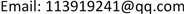191245部队，辽宁 葫芦岛

291899部队，辽宁 葫芦岛

3海军航空大学，航空基础学院，山东 烟台1. 引言

2. 故障模式识别

3. 数据代表点集

1) P ⊆ X ;

2) ∀ p ∈ P , N ε ( p , X ) ≤ q ;

3) ∀ x ∈ X ,   ∃ p ∈ P ,   satisfy   x ∈ V ε ( p ) 。

Set   X u n = X ,   P = φ While   X u n ≠ Φ , do                 Arbitrarily   select   a   x ∈ X u n                 If   N ε ( x , P ) ≤ q ,   set                 − P = P + { x }                 − X u n = X u n − { x }                 End   { if } End   { while } (1)

4. 数据特征提取

1) 选取适当的小波包函数，选取sym7小波；

2) 确定分解层数L，本文 L ≥ 5 ；

3) 根据先验知识和每个序列的起始频率，计算出位于第L层的某几个频段内的频率成分；

4) 对数据进行逐层小波包分解。分解第l层时得到位于不同频段的 2 l − 1 组序列。每组序列分别由低通滤波结果 W 2 n l 和高通滤波结果 W 2 n + 1 l 组成。

5) 利用回复公式重构信号。

E ( j , k ) = 1 N ∑ i = 1 M [ d l ( j , k ) ] 2 ,   ( l = 1 , 2 , ⋯ , N ) (2)

E ( 0 , 0 ) = ∑ i = 1 M E i ( j , k ) (3)

e = { E 1 , E 2 , ⋯ , E M } T / E ( 0 , 0 ) (4)

5. 粗糙集与数据离散化方法

S = ( U , A , V , f ) 是一个知识表达系统，其中U表示对象的非空有限集合，称为论域； A = C ∪ D ，，C称为条件属性集，D称为决策属性集； V = ∪ a ∈ A V a ， 是属性a的值域；f表示 U × A → V 是一个信息函数，它为每个对象的每个属性赋予一个信息值，即： ∀ a ∈ A , x ∈ U , f ( x , a ) ∈ V 。具有条件属性和决策属性的知识表达系统称为决策表。两个属性集C与D之间的依赖程度 γ C ( D ) 定义为

γ C ( D ) = | p o s C ( D ) | | U | (5)

σ C D ( a ) = γ C ( D ) − γ C − { a } ( D ) (6)

6. 基于粗糙集和神经网络的故障模式识别

d ( 0 , 0 ) = d ( 5 , 0 ) + d ( 5 , 1 ) + d ( 5 , 2 ) + d ( 5 , 3 ) + d ( 5 , 4 ) + d ( 5 , 5 )     + d ( 4 , 3 ) + d ( 4 , 4 ) + d ( 4 , 5 ) + d ( 3 , 3 ) + d ( 1 , 1 ) (7)

1) 本文故障模式识别与其他方法对比

The corresponding frequency bands and energy characteristics of wavelet packet decomposition coefficients of misalignment faul

Data table of fault energy featur
xiCi故障类型
C1C2C3C4C5C10C11
x10.25750.19680.01540.47430.00020.00190.0001不对中
x20.25260.15110.01030.54920.00130.00150.0002不对中
x30.23460.49700.01320.24350.00020.00040.0001不对中
… …
x20.05090.00420.00600.00380.01410.04290.7192支座松动
x220.02340.00530.00880.00480.03060.04560.7368支座松动
x230.03370.00440.00830.00540.02840.05100.7290支座松动
… …
x580.02590.03870.00520.00960.01410.01040.0004碰摩
x590.02470.03940.00390.00710.00990.00730.0003碰摩
x600.02690.03950.00530.01120.01180.00880.0003碰摩

Optimal fault diagnosis decisio

1060
2141
3161
4321
5232
6352

Comparison of pattern recognition and other method

k-means方法75.00%51.12

2) 代表点集与原始数据特征提取模式识别对比

3) 未经特征提取与特征提取后模式识别对比

7. 总结

1) 本文设计的故障识别系统达到了很高的精度。选取了原始数据代表点集，极大的减少了计算量，同时代表点集又可以表示原始数据全部信息，可以达到很高的诊断精度。

2) 代表点集经过特征提取和粗糙集处理后，能够得到最简化的最直接的与故障相关的特征，诊断精度大为提高。并且本文故障识别系统与SVM多分类与k-means识别方法相比，计算用时与精度都有所提高。

3) 以最简化的诊断规则作为输入，带入到BP神经网络中，不能涵盖所有故障样本，此时增加条件属性数量，带入到神经网络输入中，可以提高识别断精度。

Comparison of representative point and original dat

Comparison of representative point direct input and feature extractio